# Activation Functions In Python

In this post, we will go over the implementation of Activation functions in Python.

In :
import numpy as np
import matplotlib.pyplot as plt
import numpy as np


Well the activation functions are part of the neural network. Activation function determines if a neuron fires as shown in the diagram below.

In :
from IPython.display import Image
Image(filename='data/Activate_functions.png')

Out: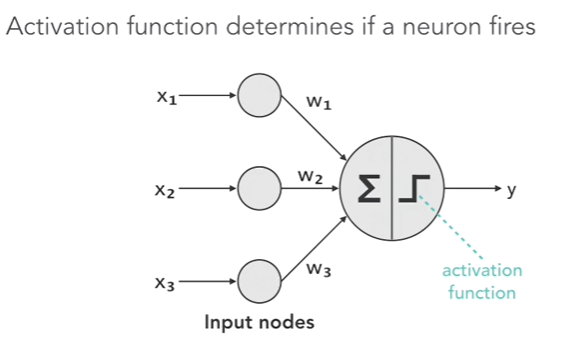## Binary Step Activation Function

Binary step function returns value either 0 or 1.

• It returns '0' if the input is the less then zero
• It returns '1' if the input is greater than zero
In :
def binaryStep(x):
''' It returns '0' is the input is less then zero otherwise it returns one '''
return np.heaviside(x,1)

In :
x = np.linspace(-10, 10)
plt.plot(x, binaryStep(x))
plt.axis('tight')
plt.title('Activation Function :binaryStep')
plt.show()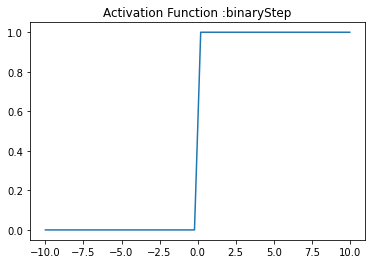## Linear Activation Function

Linear functions are pretty simple. It returns what it gets as input.

In :
def linear(x):
''' y = f(x) It returns the input as it is'''
return x

In :
x = np.linspace(-10, 10)
plt.plot(x, linear(x))
plt.axis('tight')
plt.title('Activation Function :Linear')
plt.show()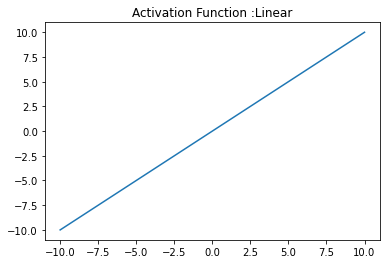## Sigmoid Activation Function

Sigmoid function returns the value beteen 0 and 1. For activation function in deep learning network, Sigmoid function is considered not good since near the boundaries the network doesn't learn quickly. This is because gradient is almost zero near the boundaries.

In :
def sigmoid(x):
''' It returns 1/(1+exp(-x)). where the values lies between zero and one '''

return 1/(1+np.exp(-x))

In :
x = np.linspace(-10, 10)
plt.plot(x, sigmoid(x))
plt.axis('tight')
plt.title('Activation Function :Sigmoid')
plt.show()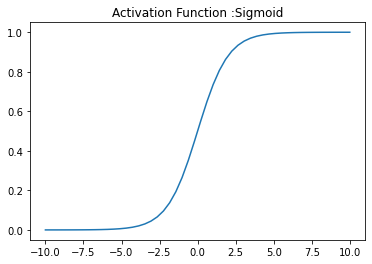## Tanh Activation Function

Tanh is another nonlinear activation function. Tanh outputs between -1 and 1. Tanh also suffers from gradient problem near the boundaries just as Sigmoid activation function does.

In :
def tanh(x):
''' It returns the value (1-exp(-2x))/(1+exp(-2x)) and the value returned will be lies in between -1 to 1.'''

return np.tanh(x)

In :
x = np.linspace(-10, 10)
plt.plot(x, tanh(x))
plt.axis('tight')
plt.title('Activation Function :Tanh')
plt.show()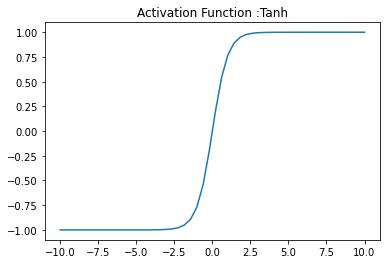## RELU Activation Function

RELU is more well known activation function which is used in the deep learning networks. RELU is less computational expensive than the other non linear activation functions.

• RELU returns 0 if the x (input) is less than 0
• RELU returns x if the x (input) is greater than 0
In :
def RELU(x):
''' It returns zero if the input is less than zero otherwise it returns the given input. '''
x1=[]
for i in x:
if i<0:
x1.append(0)
else:
x1.append(i)

return x1

In :
x = np.linspace(-10, 10)
plt.plot(x, RELU(x))
plt.axis('tight')
plt.title('Activation Function :RELU')
plt.show()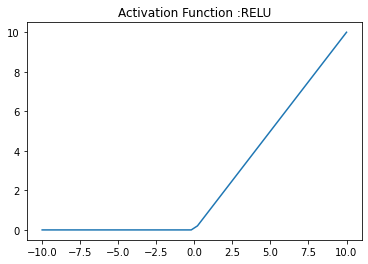## Softmax Activation Function

Softmax turns logits, the numeric output of the last linear layer of a multi-class classification neural network into probabilities.

We can implement the Softmax function in Python as shown below.

In :
def softmax(x):
''' Compute softmax values for each sets of scores in x. '''
return np.exp(x) / np.sum(np.exp(x), axis=0)

In :
x = np.linspace(-10, 10)
plt.plot(x, softmax(x))
plt.axis('tight')
plt.title('Activation Function :Softmax')
plt.show()